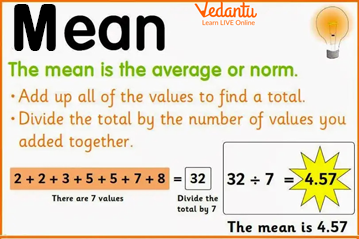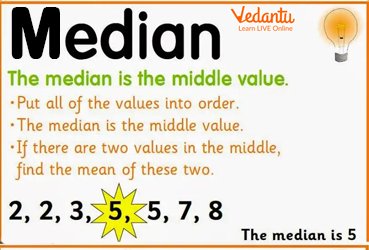Courses
Courses for Kids
Free study material
Free LIVE classes
More

# What is the Range of Data?LIVE
Join Vedantu’s FREE Mastercalss

## Introduction to Statistics

When we get a huge amount of data, we use different statistical terms to describe it. These include mean, mode, median, and range. Before calculating the range of data, it is crucial to understand these statistical terms.

So, let us discuss them more deeply.Statistics

## What is Mean?

Mean is nothing but the average of the numbers or the data given. The formula for getting mean is:

Sum of all the numbers in the data/ number of the numbers

For example: If there are five numbers from 1 to 5, their sum is 15, and the total numbers are 5. So, according to the formula, the mean is 15/5 equal to 3.Mean

## What is Mode?

Mode refers to the number that appears frequently or most of the time in a number series. If two numbers appear most frequently, there will be two modes. Hence, it will be called bimodal. If more than two numbers occur mostly, the data will be called multimodal. If all numbers appear with the same occurring frequency, then there will be no modes.Mode

## What is the Median?

Median is the middle number or a centrally occurring number in a data series. The first step to finding the median is to arrange data from lowest to highest or highest to lowest. If there are an odd number of data points, there will be one middle number as the median. Further, in the case of an even number of data points, there will be two middle numbers, and the median will be calculated by adding those numbers and dividing them by 2.Median

## What is the Range of Data?

In range statistics, the range is defined as the difference between the highest and lowest number in a data set. For example: if there is a data set given (2, 4, 6, 8, 12), the range will be 12 - 2 = 10. Therefore, the range could also be calculated by taking out the difference between the highest and lowest observation. The result is known as the range of the observation. It shows the spread of observation in statistics or data handling.Range

## What is the Range of Data Formula?

The formula to calculate the range of a set relies on the difference between the highest and the lowest values. Here are some of the range formulas.

1. Range = Highest value- lowest value.

2. Range = Highest observation- lowest observation.

3. Range = Maximum value- minimum value.

## How to Find the Range of Data?

The first step to begin finding a range involves arranging the given data sets or numbers in ascending order. This means writing the lowest value first and moving on to the highest value at last. Then, use the formula to get the range of the data set.

## Examples

Q1. Find the range of the given number series: 30, 25, 20, 35, 15, 40.

Ans: First arrange the numbers in ascending order. The new series will be; 15, 20, 25, 30, 35, and 40. Now, the highest value is 40, and the lowest value is 15. So, we can apply the formula, Range = Highest value - lowest value. Range = 40-15 = 25.

Q2. Find the range of the data series: 5, 9, 7, 11.

Ans: The new series is 5, 7, 9, and 11. The highest number is 11 and the lowest is 5. Hence, the range will be the highest number - lowest number or 11 - 5 = 6.

Q3.  What is the range of the given series: 40, 45, 55, 50?

Ans: The new series is 40, 45, 50, and 55. The highest number is 55 and the lowest number is 40. The range is the highest number- lowest number= 15.

## Practise Questions

Q1. What is the range of the series: 1, 3, 5, 7?

Ans: 6

Q2. Find the range of 2, 5, 8, and 11.

Ans: 9

Q3. What is the range of 20, 24, 28, and 32?

Ans: 12

## Summary

In Mathematics, the mean is the average of the given numbers calculated by adding the numbers and dividing them by total numbers. Mode is the most frequent or repeating number in a data series. The median is the middle number in a given data set. All these terms are accompanied by 'range'. It is the difference between the maximum and minimum numbers or highest and lowest observations.

Last updated date: 19th Sep 2023
Total views: 122.7k
Views today: 3.22k

## FAQs on What is the Range of Data?

1. Write the difference between range and mean.

The differences between range and mean are as follows.

• The range displays the spreading of observations, whereas the mean displays the average value of a data set.

• The range is equal to the difference between the highest and the lowest values, while the mean is equal to the sum of observations divided by the number of observations.

• The mathematical formula of the range is; highest number - lowest number or highest observation- lowest observation. Whereas, the mathematical formula for calculating the mean is; the sum of all numbers/the number of numbers.

2. What is the method to find the range of a data series?

To find a range of a data series follow the given steps.

Step 1: Find the highest and lowest number.

Step 2: Arrange the numbers in ascending order. This means writing the smallest number first and following a trend of moving to increase numbers. With this, the highest number will be at the end.

Step 3: Take the difference between the highest and lowest number or subtract them. The answer obtained will be the range of the given data series.